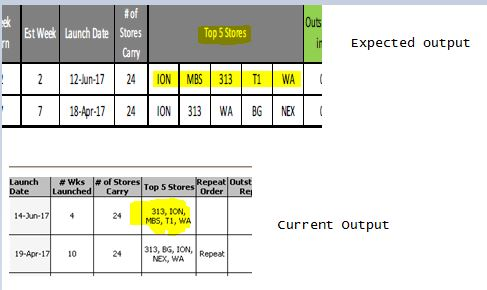# New to QlikView

Discussion board where members can get started with QlikView.

Highlighted
Honored Contributor II

## Rank order with Concatenation?

Hi All,

Using below expression able to get top 5 Store Name in straight table, Is it possible to rank the store names according to highest sold/sales instead of alphabetical order? Thanks

=Concat(DISTINCT Aggr(If(Rank(Sum(SoldQty),4) <=5, StoreName), ArticleNo, StoreName), ', ')Best Regards,

Deva

Tags (1)
1 Solution

Accepted Solutions
Honored Contributor III

## Re: Rank order with Concatenation?

Hi,

try

=Concat(DISTINCT Aggr(If(Rank(Sum(SoldQty),4) <=5, StoreName), ArticleNo, StoreName), ', ',Aggr(-Sum(SoldQty),ArticleNo,StoreName))

Regards,

Antonio

2 RepliesNot applicable

## Re: Rank order with Concatenation?

Hi,

For top 5 stores give 5 different expressions each one for first,second,third,4th and 5th shops.

for First store expression will be =Concat(DISTINCT Aggr(If(Rank(Sum(SoldQty),4) =1, StoreName), ArticleNo, StoreName))

2nd store =Concat(DISTINCT Aggr(If(Rank(Sum(SoldQty),4) =2, StoreName), ArticleNo, StoreName))

3rd store =Concat(DISTINCT Aggr(If(Rank(Sum(SoldQty),4) =3, StoreName), ArticleNo, StoreName))

4th store =Concat(DISTINCT Aggr(If(Rank(Sum(SoldQty),4) =4, StoreName), ArticleNo, StoreName))

5th store -=Concat(DISTINCT Aggr(If(Rank(Sum(SoldQty),4) =5, StoreName), ArticleNo, StoreName))

Honored Contributor III

## Re: Rank order with Concatenation?

Hi,

try

=Concat(DISTINCT Aggr(If(Rank(Sum(SoldQty),4) <=5, StoreName), ArticleNo, StoreName), ', ',Aggr(-Sum(SoldQty),ArticleNo,StoreName))

Regards,

Antonio